## Tutorial 3:  Rasch Model for Dichotomous Data(2013-7-31)

Home
1. Install R
2. Install TAM
3. Rasch Model
4. CTT, Fit
5. Partial Credit Model
6. Population Model
7. DIF

More resources

## Summary of Tutorial

This tutorial shows how to load the TAM program, read a CSV data file and fit a Rasch model for dichotomously scored data using marginal maximum likelihood estimation method. The tutorial also shows how TAM output is accessed and how the results from TAM can be further analysed in R.

## Data files

The item response data come from a mathematics test for Year 7 students. There are 15 questions. Some are multiple-choice and some are constructed-response. 876 students took the test. The data file has already been scored (data consist of 0 and 1). The test paper and the data file can be downloaded through the following links:

Numeracy test paper
Numeracy data file in csv format

An excerpt of the data file is shown below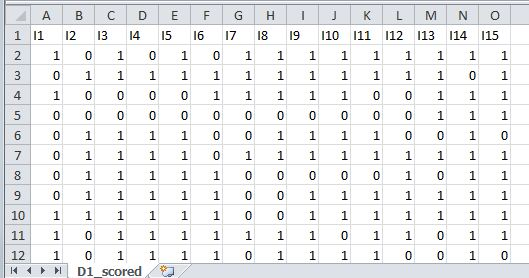The first row specifies the item names. As a rule, you should always provide item names, even for simulated data. After the header row, each row shows the item scores of each student. There is no student identifier in this data file.

## R script file

Before the TAM functions can be called, the TAM library has to be loaded into the R workspace, as shown in line 2 of the R script.
library("TAM")

Each time R is launched, the TAM library needs to be loaded into the workspace in order for TAM to run. In contrast, the installation of the seven packages as shown in Tutorial 2 only needs to be done once.

Note that R is case-sensitive, so be particularly careful with lower- and upper-case letters.

Before reading the data file, it is often helpful to set the working directory using "setwd". Once the working directory is set, all files for input and output will be accessed in the working directory unless otherwise specified. See line 5. Note that forward slash ("/") is used instead of "\". Of course, you will need to change the path in line 5 to the folder on your computer.
setwd("C:/G_MWU/TAM/Tutorials/data")

Line 6 shows the R script for reading a CSV file.
Note that the full directory path for the CSV file is not speficied in line 6, since this file is in the working directory, as set by the command in line 5. The data in the CSV file is read into a variable named D1. Run lines 5-6.

To check that the data have been read into the variable D1 correctly, in the Console window you can type "D1" followed by "Enter" to check the content of D1. The following shows the Console window in R Studio.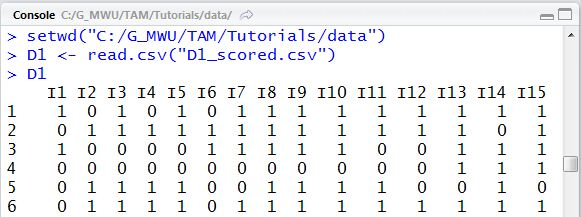If D1 does not contain the correct data, check that the file name and path are correctly specified (lower and upper case), and check that lines 5 and 6 have actually been RUN.

### Run IRT analysis

Once the item response data are read into D1, we can call the tam function for MML estimation as shown in line 9 in the R command window. Note that while the package is called TAM, the function call is lower-case "tam".
mod1 <- tam(D1)

Line 9 calls the TAM function "tam" with D1 as an argument, then assigns the results of the IRT analysis to an object called mod1. When line 9 is run, intermediate results of estimation are displayed in the Console window, as shown below.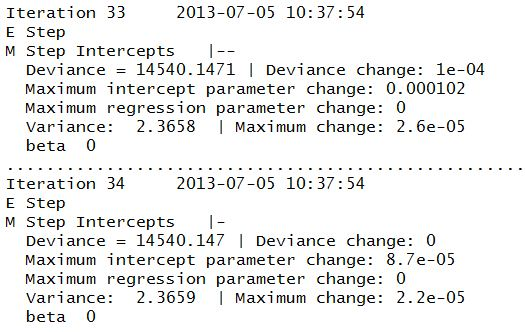The run took 34 iterations to converge with an elapsed time of just a fraction of a second.

### Get IRT results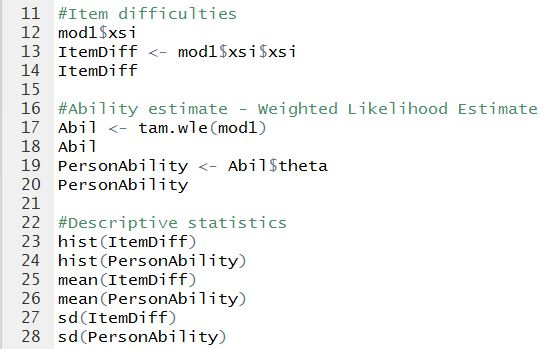The results of the IRT run are encapsulated in an object called "mod1" (see line 9). To access specific results of the analysis, the syntax is "mod1\$" followed by a variable name. For example, to get estimated item difficulties, type "mod1\$xsi".

You can refer to the TAM.pdf document for the names of variables to extract the results. In the specification for the function "tam" in the pdf reference manual, under the heading of "Value", variables holding the IRT results are listed. These variables can all be accessed using the form "mod1\$xyz" where xyz is a variable in the "Value" list.

Line 12 asks for a display of the content of "xsi" from the IRT analysis. In the Console window, the following is displayed.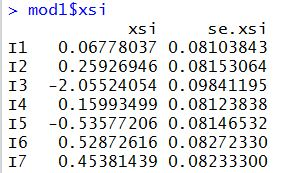If you only want to extract the item difficulties (xsi) and not the standard errors, you can type "mod1\$xsi\$xsi". This means the "xsi" variable in the "mod1\$xsi" object. See lines 13 and 14.

To get person ability estimates, the function "tam.wle" needs to be called. Under the MML model, person abilities are not part of the IRT model. But weighted likelihood estimate of ability can be estimated given the item difficulties obtained in the IRT run. Lines 17-20 show the computation of ability estimates.

When line 17 is run, the Console window shows the following output.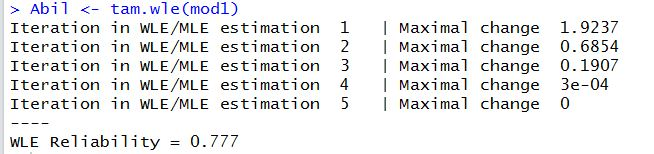The results of the "tam.wle" function are stored in an object call "Abil" (see lines 17 and 18). This object has a number of sub-variables in it, as shown below.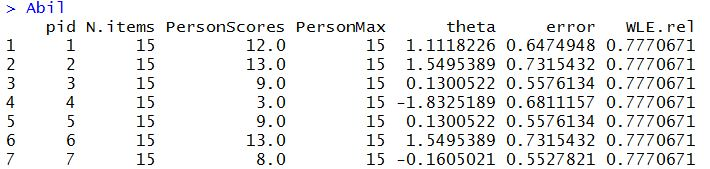To extract just the WLE estimates and not the other information for each respondent, use "Abil\$theta". See lines 19 and 20.

Under TAM, the default origin (i.e., zero) of the ability scale is set as the mean ability. In ConQuest terminology, this is "set constraint=cases".

### Analyse TAM results with R base functions

As the IRT results from TAM are stored in R variables, R base functions can be applied to these variables. For example, "hist" is an R base function for histogram. hist(ItemDiff) will show a histogram of item difficulties. hist(PersonAbility) will show a histogram of WLE abilities (see lines 23 and 24), as shown below.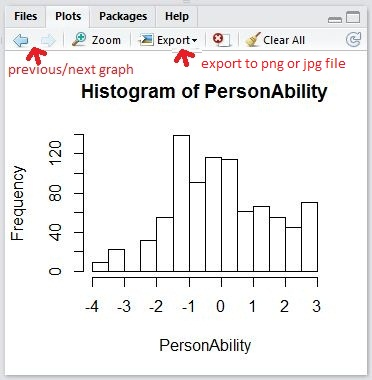Similarly, mean(ItemDiff) will give the mean of the item difficulties. sd(ItemDiff) will give the standard deviation.

### Extension

The following R code can be used to plot the histograms of ability and item difficulty on the same graph. The two histograms will be in a new window (not part of R Studio). Plotting both histograms on the same graph allows for a comparison between item difficulties and abilities, so that test targetting can be evaluated.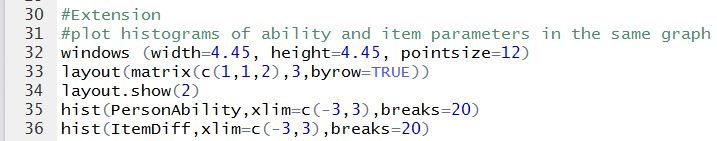It can be seen that for this example, the items are relatively easy for the students.